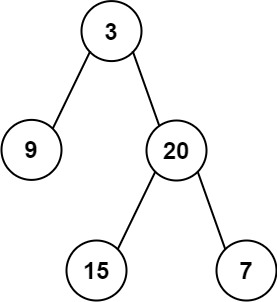106. Construct Binary Tree from Inorder and Postorder Traversal
Medium
6.9K
104

Given two integer arrays `inorder` and `postorder` where `inorder` is the inorder traversal of a binary tree and `postorder` is the postorder traversal of the same tree, construct and return the binary tree.

Example 1:```Input: inorder = [9,3,15,20,7], postorder = [9,15,7,20,3]
Output: [3,9,20,null,null,15,7]
```

Example 2:

```Input: inorder = [-1], postorder = [-1]
Output: [-1]
```

Constraints:

• `1 <= inorder.length <= 3000`
• `postorder.length == inorder.length`
• `-3000 <= inorder[i], postorder[i] <= 3000`
• `inorder` and `postorder` consist of unique values.
• Each value of `postorder` also appears in `inorder`.
• `inorder` is guaranteed to be the inorder traversal of the tree.
• `postorder` is guaranteed to be the postorder traversal of the tree.
Accepted
517.6K
Submissions
863.5K
Acceptance Rate
59.9%

Seen this question in a real interview before?
1/4
Yes
No

Discussion (0)

Related Topics